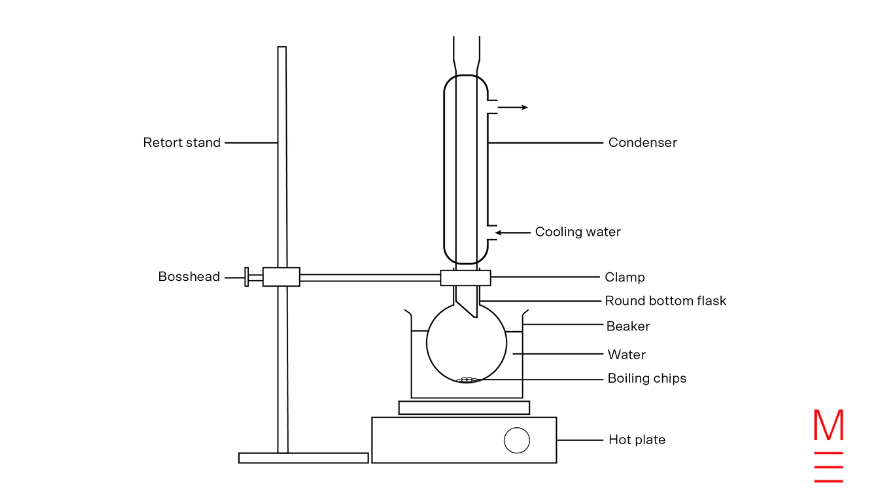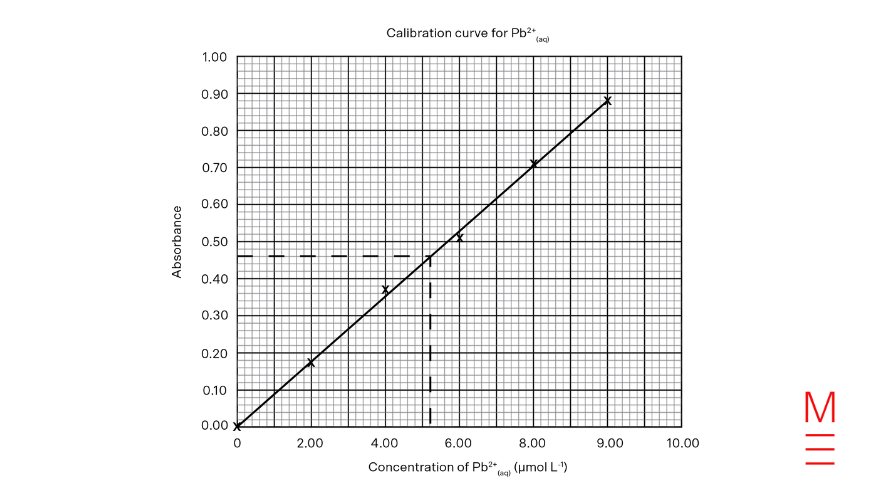# Essential HSC Chemistry Exam Techniques

The following essential HSC Chemistry Exam Techniques have been compiled from the NESA website and the 2016 Term 1 Matrix Education Year 12 Chemistry Theory Book.

## Calculations

• Start your calculations with a balanced chemical equation
• Check your data is in standard units before substituting them into a formula
• Set working out clearly since marks are awarded for working out and your final answer.
• Round off your answers at the end of your calculation, instead of during the calculation. Keep a few more significant figures than you need during the calculations (2-3 additional digits is a good rule of thumb)
• Ensure you give your final answer to the correct number of significant figures. To work out the number of significant figures for HSC questions:
1. Going from left to right, the first non-zero digit is the first significant figure
2. Each digit to its right is a significant figure.
For example: 10.0 counts as 3 significant figure as the first non-zero digit is 1 and the next 2 digits to its right is a significant figure. However, 0.50 has 2 significant figures as the first non-zero digit is 5, with the 0 following it making up the second significant figure.
• Know how to change the figures that appear on your calculator screen to scientific notation. For example, 1.0 x 10-10  is scientific notation but 10-10  is not.
• Check your answer makes sense! For example, a pH value of 995 does not make sense.

## DiagramDiagram of Reflux Equipment

• Draw the diagram in pencil as it can be easily erased.
• Label your diagrams clearly and thoroughly
• Simple 2-D diagrams are best and unless requested, 3-D diagrams are usually unnecessary
• Make sure your image is large enough to be clear
• Include a diagram as part of your response to clarify your answer or if it is requested.

## Graphs

• Place the independent variable on the x-axis (horizontal) and the dependent variable on the y-axis (vertical).
• Choose your scale so it best fits the range of each variable and it covers as much of the provided space as possible. The scale on the x-axis and y-axis do not need to match.
• Make sure you label axes clearly, including units, if appropriate.
• Mark each data point with a cross (“x”). Use a clear ruler to help you plot points that are far from the axes.
• Draw a smooth line of best fit, ignoring any outliers. A clear ruler can be useful to draw a line of best fit for a linear trend as you can see the data points beneath it. Remember that a line of best fit does not necessarily have to pass through the origin.
• If you are asked to determine a value by extrapolating or interpolating from a graph, show working on the graph by using a dotted line.
• Provide a title for your graph, which states what it’s about.## Long Response

• Familiarise yourself with the NESA Glossary of Key Words. These will help you understand what the questions require. For example, ‘evaluate’ means that you need to describe the issue and criteria, list the advantages and disadvantages, and make a judgement based on these.
• Your answers can include dot points, diagrams, tables, subheadings, and a balanced chemical equation if applicable.
• Your answer should be logical, structured and relevant to the questions asked. Read the question carefully so you know exactly what they’re asking and take some time to plan your response. Statements that do not answer the question will not score marks.
• Ensure you don’t provide any information that contradicts potentially correct information provided earlier. A contradiction cancels out the mark you may have otherwise been awarded.
• Don’t simply reword information given in the question.

## First-Hand Investigations

• If you are asked about an investigation that you haven’t personally performed, pretend you have done the experiment and answer the questions based on your theoretical knowledge.
• When writing a procedure, specify equipment and realistic quantities of reagents.
• Understand why each step of the procedure is performed and be aware of potential sources of error.
• If the question asks for a safety assessment, give a specific safety measure that relates to the investigation, rather than generalised safety measures.
• Understand these concepts:
• Independent and dependent variables
• Controlled variables and why they are necessary
• Qualitative and quantitative measurements
• Destructive and non-destructive techniques
• Validity, reliability and accuracy. Our ‘6 Common Mistakes HSC Physics Students Make In Exams‘ article outlines the differences between the three.
• Ways of improving validity, reliability and accuracy

## General Exam

• Use the mark allocation and the amount of space provided in the answer book as a guide to how much you should write or draw. Be aware that a longer response will not automatically result in higher marks, writing an unnecessarily long answer uses up valuable time that could be spent on other questions and irrelevant extraneous information may be penalised.
• Where possible, include balanced chemical equations in your answers with states (e.g. s, aq, g etc.)
• Use specific language in your answers e.g. “increased the concentration of HCl”, not “affected the concentration of HCl”.

,

#### Related Posts

Platform Slot Gacor CMS Checker